# Side Window Filtering 论文解读和C++实现

Side Window Filtering

side window 盒子滤波C++复现代码：

Ldpe2G/ArmNeonOptimization

side window 中值滤波C++复现代码：

Ldpe2G/ArmNeonOptimization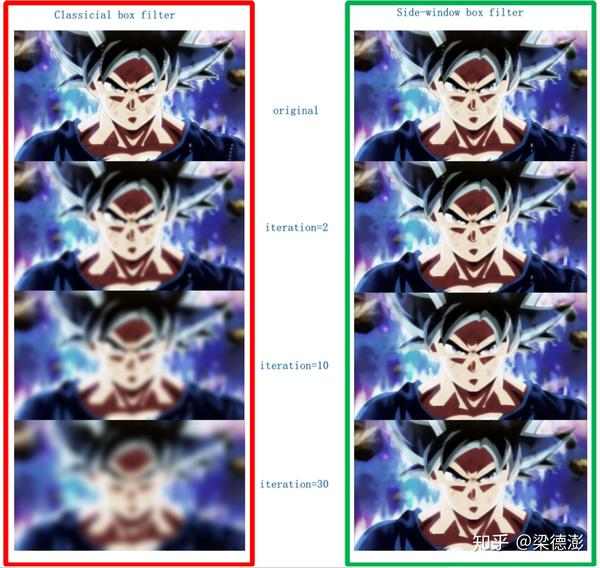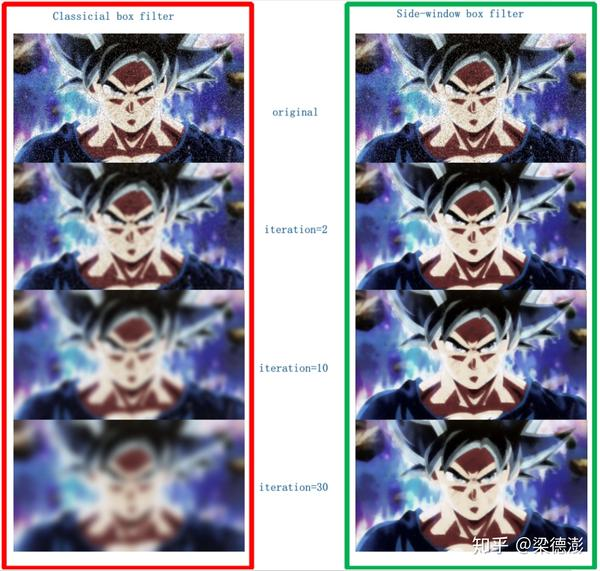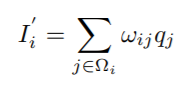Ω是以像素点 i 为中心的滤波窗口，w是滤波权值，q是原图像素值，I'是输出结果。但是这样以一个像素为中心去滤波会导致的问题是，如果一个像素点处在边缘位置（这里的边缘不是指图片的大小边界，而是指图像中物体的边缘）的话，以像素为中心去滤波会导致滤波结果的边缘部分变模糊。具体是为什么，论文中给出了分析过程。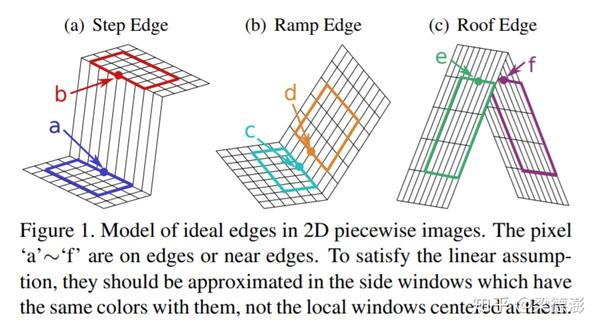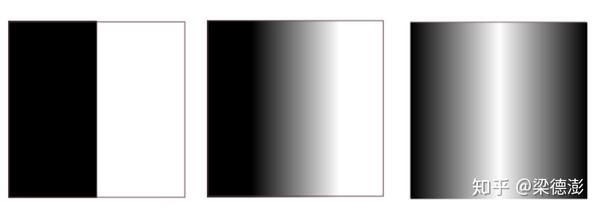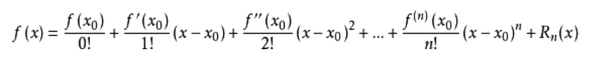“泰勒公式是将一个在 x=x0 处具有n阶导数的函数 f(x) 利用关于 (x - x0) 的n次多项式来逼近函数的方法。”----百度百科

g(x - 2ε, y) ≈ f(x0) + f'(x0)(x - x0)

= g(x - ε, y) + g'(x - ε, y)(x - 2ε - (x - ε))

= g(x - ε, y) + g'(x - ε, y)(- ε)

g(x + 2ε, y) ≈ f(x0) + f'(x0)(x - x0)

= g(x + ε, y) + g'(x + ε, y)(x + 2ε - (x + ε))

= g(x + ε, y) + g'(x + ε, y)ε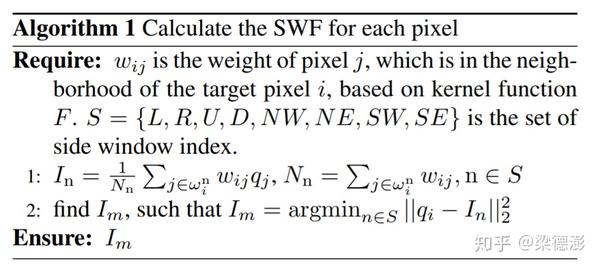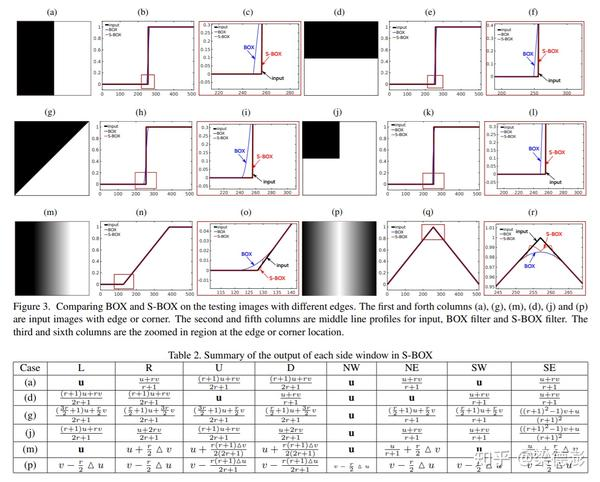​ 熊猫宝宝原图​ 滤波结果, box filter, iteration = 10​ 滤波结果, side window box filter, iteration = 10​ 熊猫宝宝原图+椒盐噪声​ 去噪结果, box filter, iteration = 10​ 去噪结果, side window box filter, iteration = 10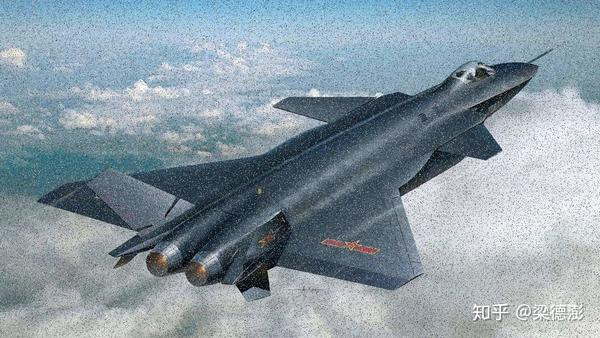​ 歼20+椒盐噪声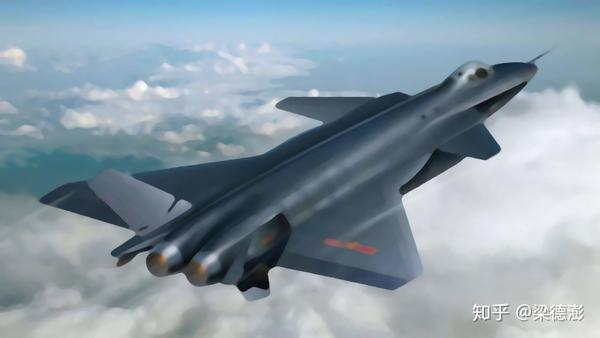​ 去噪结果, median filter, iteration = 10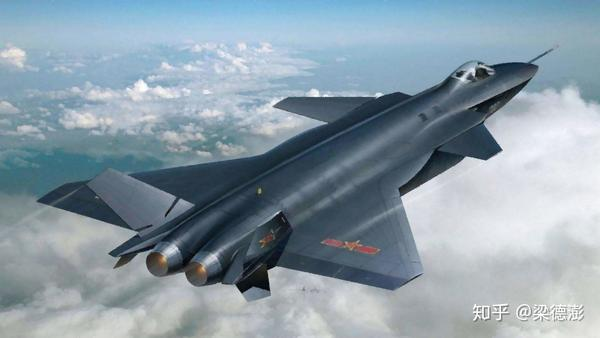​ 去噪结果, side window median filter, iteration = 10